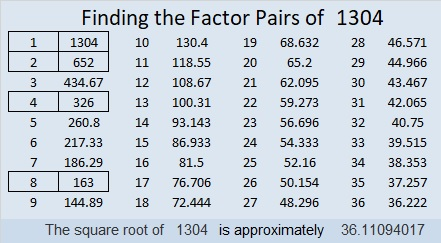# 1304 and Level 3

Which factor pairs of 45 and 18 have only numbers from 1 to 10 in them? Answer that question, put the factors in the appropriate places, and then work your way down this level 3 puzzle cell by cell until you’ve solved it.Print the puzzles or type the solution in this excel file: 10-factors-1302-1310

Here are a few facts about the number 1304:

• 1304 is a composite number.
• Prime factorization: 1304 = 2 × 2 × 2 × 163, which can be written 1304 = 2³ × 163
• The exponents in the prime factorization are 3 and 1. Adding one to each and multiplying we get (3 + 1)(1 + 1) = 4 × 2 = 8. Therefore 1304 has exactly 8 factors.
• Factors of 1304: 1, 2, 4, 8, 163, 326, 652, 1304
• Factor pairs: 1304 = 1 × 1304, 2 × 652, 4 × 326, or 8 × 163
• Taking the factor pair with the largest square number factor, we get √1304 = (√4)(√326) = 2√326 ≈ 36.110941304 is the difference of two squares two ways:
327² – 325² = 1304
165² – 161² = 1304

This site uses Akismet to reduce spam. Learn how your comment data is processed.